# NCERT Solutions For Class 9 Math Chapter – 7 Exercise – 7.4

NCERT Solutions For Class 9 Math Chapter – 7 Exercise – 7.4

Q1. Show that in a right angled triangle the hypotenuse is the longest side.

Solution:-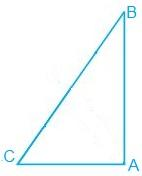ABC is a right angled at A.

∟A > ∟B and ∟A > ∟C.

BC > AC and BC > AB [ Because the side opposite to the greater angle is longer.]

BC is the longest side.

The hypotenuse is the largest side in an right angled triangle.

Q2. In figure sides AB and AC of ∆ABC are extended to points P and Q respectively. Also , ∟PBC < ∟QCB. Show that AC > AB.

Solution:-

src="https://cdn1.byjus.com/wp-content/uploads/2020/07/ncert-solutions-class-9-chapter-7-23.png" alt="Ncert solutions class 9 chapter 7-23" />

∟PBC < ∟QCB.

1800 – ∟ABC <1800 – ∟ACB.

= – ∟ABC < – ∟ACB.

= ∟ABC > ∟ACB

AC > AB.

1. In Fig. 7.49, B < A and C < D. Show that AD < BC.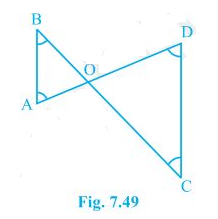Solution:

In the question, it is mentioned that angles B and angle C is smaller than angles A and D, respectively i.e. B < A and C < D.

Now,

Since the side opposite to the smaller angle is always smaller

AO < BO — (i)

And OD < OC —(ii)

By adding equation (i) and equation (ii) we get

AO+OD < BO + OC

1. AB and CD are respectively the smallest and longest sides of a quadrilateral ABCD (see Fig. 7.50).

Show that A > C and B > D.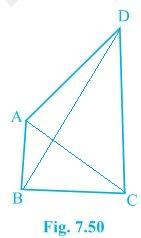Solution:

In ΔABD, we see that

So, ADB < ABD — (i) (Since angle opposite to longer side is always larger)

Now, in ΔBCD,

BC < DC < BD

Hence, it can be concluded that

BDC < CBD — (ii)

Now, by adding equation (i) and equation (ii) we get,

ADB + BDC < ABD + CBD

B > D

Similarly, In triangle ABC,

ACB < BAC — (iii) (Since the angle opposite to the longer side is always larger)

DCA < DAC — (iv)

By adding equation (iii) and equation (iv) we get,

ACB + DCA < BAC+DAC

∴ A > C

1. In Fig 7.51, PR > PQ and PS bisect QPR. Prove that PSR > PSQ.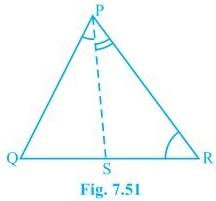Solution:

It is given that PR > PQ and PS bisects QPR

Now we will have to prove that angle PSR is smaller than PSQ i.e. PSR > PSQ

Proof:

QPS = RPS — (ii) (As PS bisects ∠QPR)

PQR > PRQ — (i) (Since PR > PQ as angle opposite to the larger side is always larger)

PSR = PQR + QPS — (iii) (Since the exterior angle of a triangle equals to the sum of opposite interior angles)

PSQ = PRQ + RPS — (iv) (As the exterior angle of a triangle equals to the sum of opposite interior angles)

PQR +QPS > PRQ +RPS

Thus, from (i), (ii), (iii) and (iv), we get

PSR > PSQ

1. Show that of all line segments drawn from a given point not on it, the perpendicular line segment is the shortest.

Solution:

First, let “l” be a line segment and “B” be a point lying on it. A line AB perpendicular to l is now drawn. Also, let C be any other point on l. The diagram will be as follows: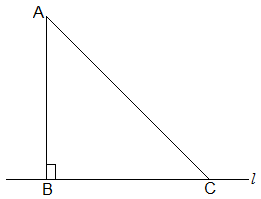To prove:

AB < AC

Proof:

In ΔABC, B = 90°

Now, we know that

A+B+C = 180°

∴ A +C = 90°

Hence, C must be an acute angle which implies C < B

So, AB < AC (As the side opposite to the larger angle is always larger)